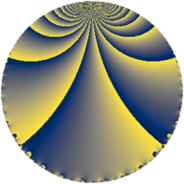# Properties

 Label 128.2.b.bLevel $128$ Weight $2$ Character orbit 128.b Analytic conductor $1.022$ Analytic rank $0$ Dimension $2$ CM discriminant -4 Inner twists $4$

# Related objects

## Newspace parameters

 Level: $$N$$ $$=$$ $$128 = 2^{7}$$ Weight: $$k$$ $$=$$ $$2$$ Character orbit: $$[\chi]$$ $$=$$ 128.b (of order $$2$$, degree $$1$$, minimal)

## Newform invariants

 Self dual: no Analytic conductor: $$1.02208514587$$ Analytic rank: $$0$$ Dimension: $$2$$ Coefficient field: $$\Q(\sqrt{-1})$$ Defining polynomial: $$x^{2} + 1$$ Coefficient ring: $$\Z[a_1, \ldots, a_{5}]$$ Coefficient ring index: $$2^{2}$$ Twist minimal: yes Sato-Tate group: $\mathrm{U}(1)[D_{2}]$

## $q$-expansion

Coefficients of the $$q$$-expansion are expressed in terms of $$i = \sqrt{-1}$$. We also show the integral $$q$$-expansion of the trace form.

 $$f(q)$$ $$=$$ $$q + 4 i q^{5} + 3 q^{9} +O(q^{10})$$ $$q + 4 i q^{5} + 3 q^{9} -4 i q^{13} -2 q^{17} -11 q^{25} -4 i q^{29} -12 i q^{37} + 10 q^{41} + 12 i q^{45} -7 q^{49} + 4 i q^{53} + 12 i q^{61} + 16 q^{65} + 6 q^{73} + 9 q^{81} -8 i q^{85} -10 q^{89} -18 q^{97} +O(q^{100})$$ $$\operatorname{Tr}(f)(q)$$ $$=$$ $$2q + 6q^{9} + O(q^{10})$$ $$2q + 6q^{9} - 4q^{17} - 22q^{25} + 20q^{41} - 14q^{49} + 32q^{65} + 12q^{73} + 18q^{81} - 20q^{89} - 36q^{97} + O(q^{100})$$

## Character values

We give the values of $$\chi$$ on generators for $$\left(\mathbb{Z}/128\mathbb{Z}\right)^\times$$.

 $$n$$ $$5$$ $$127$$ $$\chi(n)$$ $$-1$$ $$1$$

## Embeddings

For each embedding $$\iota_m$$ of the coefficient field, the values $$\iota_m(a_n)$$ are shown below.

For more information on an embedded modular form you can click on its label.

Label $$\iota_m(\nu)$$ $$a_{2}$$ $$a_{3}$$ $$a_{4}$$ $$a_{5}$$ $$a_{6}$$ $$a_{7}$$ $$a_{8}$$ $$a_{9}$$ $$a_{10}$$
65.1
 − 1.00000i 1.00000i
0 0 0 4.00000i 0 0 0 3.00000 0
65.2 0 0 0 4.00000i 0 0 0 3.00000 0
 $$n$$: e.g. 2-40 or 990-1000 Significant digits: Format: Complex embeddings Normalized embeddings Satake parameters Satake angles

## Inner twists

Char Parity Ord Mult Type
1.a even 1 1 trivial
4.b odd 2 1 CM by $$\Q(\sqrt{-1})$$
8.b even 2 1 inner
8.d odd 2 1 inner

## Twists

By twisting character orbit
Char Parity Ord Mult Type Twist Min Dim
1.a even 1 1 trivial 128.2.b.b 2
3.b odd 2 1 1152.2.d.d 2
4.b odd 2 1 CM 128.2.b.b 2
5.b even 2 1 3200.2.d.e 2
5.c odd 4 1 3200.2.f.c 2
5.c odd 4 1 3200.2.f.d 2
8.b even 2 1 inner 128.2.b.b 2
8.d odd 2 1 inner 128.2.b.b 2
12.b even 2 1 1152.2.d.d 2
16.e even 4 1 256.2.a.b 1
16.e even 4 1 256.2.a.c 1
16.f odd 4 1 256.2.a.b 1
16.f odd 4 1 256.2.a.c 1
20.d odd 2 1 3200.2.d.e 2
20.e even 4 1 3200.2.f.c 2
20.e even 4 1 3200.2.f.d 2
24.f even 2 1 1152.2.d.d 2
24.h odd 2 1 1152.2.d.d 2
32.g even 8 4 1024.2.e.k 4
32.h odd 8 4 1024.2.e.k 4
40.e odd 2 1 3200.2.d.e 2
40.f even 2 1 3200.2.d.e 2
40.i odd 4 1 3200.2.f.c 2
40.i odd 4 1 3200.2.f.d 2
40.k even 4 1 3200.2.f.c 2
40.k even 4 1 3200.2.f.d 2
48.i odd 4 1 2304.2.a.a 1
48.i odd 4 1 2304.2.a.p 1
48.k even 4 1 2304.2.a.a 1
48.k even 4 1 2304.2.a.p 1
80.k odd 4 1 6400.2.a.l 1
80.k odd 4 1 6400.2.a.m 1
80.q even 4 1 6400.2.a.l 1
80.q even 4 1 6400.2.a.m 1

By twisted newform orbit
Twist Min Dim Char Parity Ord Mult Type
128.2.b.b 2 1.a even 1 1 trivial
128.2.b.b 2 4.b odd 2 1 CM
128.2.b.b 2 8.b even 2 1 inner
128.2.b.b 2 8.d odd 2 1 inner
256.2.a.b 1 16.e even 4 1
256.2.a.b 1 16.f odd 4 1
256.2.a.c 1 16.e even 4 1
256.2.a.c 1 16.f odd 4 1
1024.2.e.k 4 32.g even 8 4
1024.2.e.k 4 32.h odd 8 4
1152.2.d.d 2 3.b odd 2 1
1152.2.d.d 2 12.b even 2 1
1152.2.d.d 2 24.f even 2 1
1152.2.d.d 2 24.h odd 2 1
2304.2.a.a 1 48.i odd 4 1
2304.2.a.a 1 48.k even 4 1
2304.2.a.p 1 48.i odd 4 1
2304.2.a.p 1 48.k even 4 1
3200.2.d.e 2 5.b even 2 1
3200.2.d.e 2 20.d odd 2 1
3200.2.d.e 2 40.e odd 2 1
3200.2.d.e 2 40.f even 2 1
3200.2.f.c 2 5.c odd 4 1
3200.2.f.c 2 20.e even 4 1
3200.2.f.c 2 40.i odd 4 1
3200.2.f.c 2 40.k even 4 1
3200.2.f.d 2 5.c odd 4 1
3200.2.f.d 2 20.e even 4 1
3200.2.f.d 2 40.i odd 4 1
3200.2.f.d 2 40.k even 4 1
6400.2.a.l 1 80.k odd 4 1
6400.2.a.l 1 80.q even 4 1
6400.2.a.m 1 80.k odd 4 1
6400.2.a.m 1 80.q even 4 1

## Hecke kernels

This newform subspace can be constructed as the kernel of the linear operator $$T_{3}$$ acting on $$S_{2}^{\mathrm{new}}(128, [\chi])$$.

## Hecke characteristic polynomials

$p$ $F_p(T)$
$2$ $$T^{2}$$
$3$ $$T^{2}$$
$5$ $$16 + T^{2}$$
$7$ $$T^{2}$$
$11$ $$T^{2}$$
$13$ $$16 + T^{2}$$
$17$ $$( 2 + T )^{2}$$
$19$ $$T^{2}$$
$23$ $$T^{2}$$
$29$ $$16 + T^{2}$$
$31$ $$T^{2}$$
$37$ $$144 + T^{2}$$
$41$ $$( -10 + T )^{2}$$
$43$ $$T^{2}$$
$47$ $$T^{2}$$
$53$ $$16 + T^{2}$$
$59$ $$T^{2}$$
$61$ $$144 + T^{2}$$
$67$ $$T^{2}$$
$71$ $$T^{2}$$
$73$ $$( -6 + T )^{2}$$
$79$ $$T^{2}$$
$83$ $$T^{2}$$
$89$ $$( 10 + T )^{2}$$
$97$ $$( 18 + T )^{2}$$